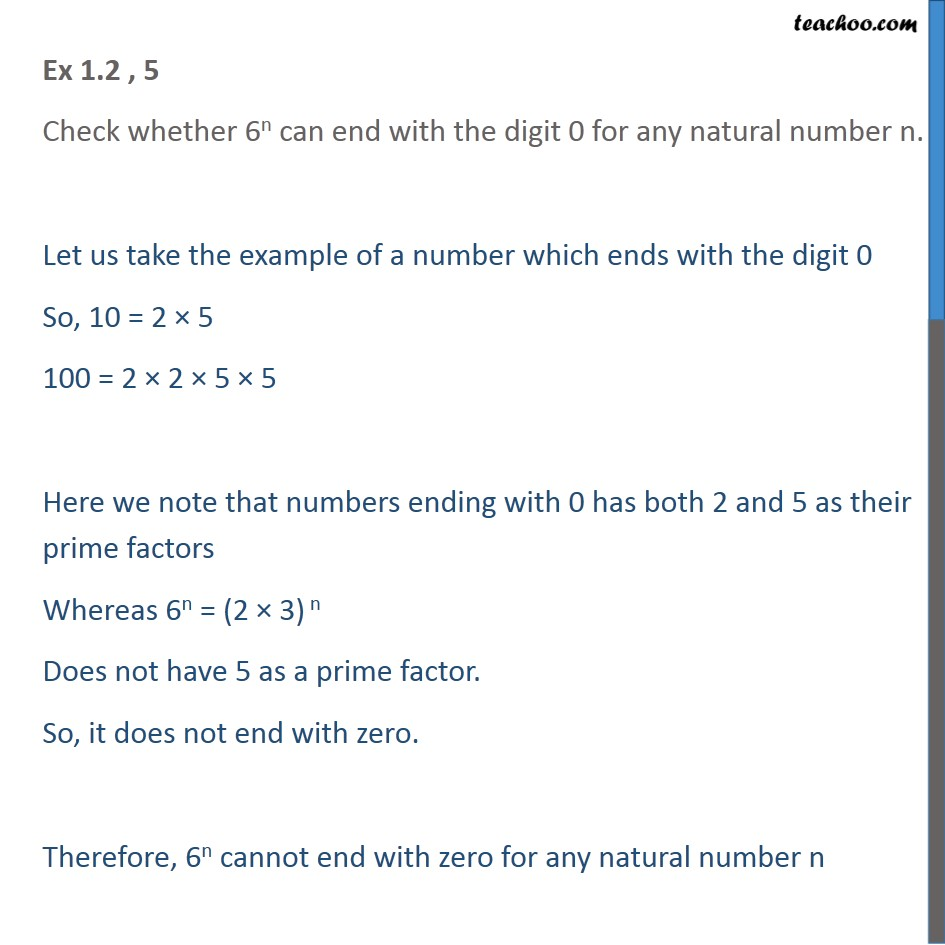1. Chapter 1 Class 10 Real Numbers (Term 1)
2. Serial order wise
3. Ex 1.2

Transcript

Ex 1.2 , 5 Check whether 6n can end with the digit 0 for any natural number n. Let us take the example of a number which ends with the digit 0 So, 10 = 2 5 100 = 2 2 5 5 Here we note that numbers ending with 0 has both 2 and 5 as their prime factors Whereas 6n = (2 3) n Does not have 5 as a prime factor. So, it does not end with zero. Therefore, 6n cannot end with zero for any natural number n

Ex 1.2

Chapter 1 Class 10 Real Numbers (Term 1)
Serial order wise